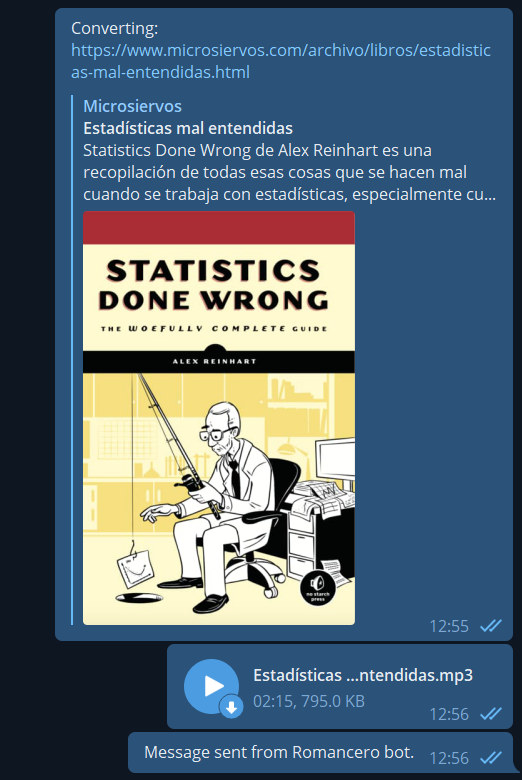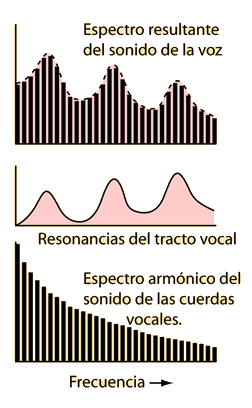## Romancero bot: converting article text into voice with Amazon Polly and sending it to Telegram

The following Python script extracts the content of an article using Readibility (ported to Python), converts it to voice using the Amazon Polly service and finally sends the audio as a voice note to a given user in Telegram using Telethon (Telegram client for Python).

For running the script you will need to install the following Python packages:

Also, you will need to create a AWS account. If you already have an AWS account, make sure that you have a user created in the IAM Management Console with the following permissions:

When creating this user, make sure you write down its ID access key and its secret access key. You will need them to configure the aws-cli client.

With this Amazon credentials, you can configure the AWS client by executing the following command:

In this step, you will need to fulfill the details with the user credentials you wrote down when creating it.

Now, you will need to create a Telegram API ID. For this, you can go to the Telegram section «Create an Application«. After following the steps described in the official documentation, you will obtain an API ID (it’s a number) and a API hash (it’s a string).

With these steps already completed, you can place all the needed details in the script and run it.

The user you specified will receive a message like this:## Extracción de parámetros para reconocimiento de voz

A la hora de conformar una vocal, las cuerdas vocales vibran, produciendo una vibración en el aire en forma de tren de pulsos. A su vez, este tren de pulsos pasa a través del tracto vocal, el cual, dependiendo del fonema que se ha pronunciado actuará como un filtro y modificará las componentes frecuenciales del tren de pulsos.Para cada fonema, el filtro resultante del tracto vocal es diferente. Mientras hablamos, el responsable de que una palabra o un fonema suene de una manera particular es el tracto vocal. Se puede ver un ejemplo intentando decir ‘Hola’ con la boca cerrada o sin mover la boca. Aunque las cuerdas vocales vibran de la misma manera que lo hacen cuando la boca está abierta, no se entiende bien.

Por tanto, en reconocimiento de voz es interesante poder extraer características del tracto vocal, que corresponde a la forma de la envolvente del sonido visto desde el punto de vista frecuencial. Entonces, ¿cómo extraemos la envolvente? Utilizando el análisis cepstral.

### Análisis cepstral

El cepstrum de una señal es el resultado de calcular la transformada de Fourier (FT, del inglés Fourier Transform) del espectro de la señal estudiada en escala logarítmica (dB). El nombre cepstrum deriva de invertir las cuatro primeras letras de spectrum. El cepstrum puede ser visto como una información del ritmo de cambio de las diferentes bandas de un espectro.

Wikipedia

El cepstrum o análisis cepstral aplicado a una voz equivale a:
La voz $$V\left(f\right) = P \left(f \right) \cdot T\left( f \right)$$, donde $$P \left(f \right)$$ son las componentes frecuenciales producidas por las cuerdas vocales y $$T \left(f \right)$$ es el filtro equivalente del tracto vocal.
Si aplicamos logaritmo a $$V\left( f\right)$$, tenemos $$\log{\left[ V\left( f \right) \right]} = \log{\left[ P\left( f \right) \right]} + \log{\left[ T\left( t \right) \right]}$$

La transformada de Fourier de $$T\left( f \right)$$ tiene bajas frecuencias. Por otra parte, la transformada de Fourier de $$P\left( f \right)$$ contiene altas frecuencias.
De esta manera, si volvemos a hacer la transformada de Fourier de $$V\left( f \right)$$ y nos quedamos solo con las bajas frecuencias, habremos eliminado la componente de $$P\left( f \right)$$ y tendremos solo la información de $$T\left( f \right)$$. Hay que pensar en el concepto de Transformada de Fourier de la Transformada de Fourier $$\left(FFT\left\{ FFT \left\{ x \right\} \right\} \right)$$.

Sin embargo, en audio no se utiliza exactamente de esta manera, si no que además se aplica un banco de filtros Mel y se utiliza como segunda transformada la DCT en lugar de la transformada de Fourier debido a sus propiedades de compresión de información (consigue concentrar la mayor parte de la información en pocos coeficientes). Es por ello que en la extracción de parámetros para reconocimiento de voz se utilizan los Mel Frequency Cepstral Coeﬃcients (MFCC).

### Mel Frequency Cepstral Coeﬃcients (MFCC)

Para empezar a extraer los parámetros MFCC de un archivo de audio, el audio se enventana. En lugar de extraer los parámetros MFCC de una pieza de 1 segundo (que podría ser aproximadamente la duración de una palabra), se extraen los MFCC de segmentos de 25 ms. La ventana es una ventana de tipo Hanning o Hamming para evitar los armónicos artificiales que aparecen al utilizar ventanas cuadradas. Cada ventana se desplaza 10 ms en tiempo, por lo que existe una superposición entre una ventana y otra.1. A cada una de estas ventanas se aplica la FFT.
2. A la FFT de cada ventana se le aplica el banco de filtros MEL.
3. Se calcula el logaritmo
4. Se hace la transformada discreta del coseno (DCT)
5. Nos quedamos con los N primeros coeficientes (baja frecuencia). Normalmente 12 coeficientes. O también 12 para bajas frecuencias y 1 para la energía media de la ventana.

Como ya habíamos dicho la diferencia entre los cepstrum y los MFCC, es que una vez hecho la FFT en el paso 1, se filtra mediante los filtros MEL y en lugar de aplicar en el paso 4 otra vez una FFT, se aplica la DCT .

Cabe destacar que en reconocimiento de voz no se reconocen fonemas sino palabras. Por tanto, para un archivo de audio de 1 segundo, tendremos $$\frac{1~seg-25~ms}{10~ms} = 97.5 \approx 98$$

parámetros MFCC $$\left( \left\{p_1, p_2, …, p_m \right\} \right)$$ y cada parámetro MFCC contendrá N elementos $$\left( p_m = \left\{c_1, c_2, …, c_N \right\} \right)$$.

Función para la extracción de MFCC en MATLAB de Kamil Wojcicki

Parámetros típicos de la función mfcc.m:

Si la señal por ejemplo está grabada a 16 kHz, tiene componentes frcuenciales hasta 8 kHz. 7 kHz como frecuencia superior máxima es una buena elección para no tener en cuenta las frecuencias próximas a Nyquist que suelen verse atenuadas y porque hasta 7 kHz hay suficiente información de voz. Por debajo se puede limitar a 50 Hz, dado que la voz humana no tiene apenas información por debajo y así se puede evitar ruidos.